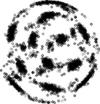<< WebHome

# LATfield2 + Gevolution tutorial

For files, see https://bitbucket.org/broukema/cosmotorun19 .

# Using the tutorial examples

## Step 0 (optional)

For those who have never coded in C++ a basic intro using three examples. Use cppBasics.cpp to gain enough familiarity with the C++ language to be able to follow the gevolution session discussions. In the main function uncomment the relevant lines to see output of the example.

• a) The <iostream> header replaces <stdio.h> for input/output
• b) C++ template. A simple template function that can receive two integers or floats and returns the maximum value of the correct data type. (Gevolution uses function and class templates to define fields such as gravitational potential, tensor fields etc on lattices, the intention is that the workshop participant recognises template notation.)
• c) A simple C++ class that has two member functions. Classes are a foreign concept for those who have only coded in C or Fortran 90.
• d) Overloaded operators. (Required if the participant wants to decode physics equations in gevolution. Only a superficial understanding is required.)

## Step 1 (Install required software)

Install gevolution, LATfield2, hdf5 and fftw3.

## Step 2 (Run potForce.cpp, dft_forwards.cpp)

Purpose: Be able to use "Lattice", "Site", "Field", "PlanFFT" classes.

(Gevolution takes Fourier transforms (computes discrete Fourier transforms) when setting up the simulation initial conditions. It uses "Lattice", "Site", "Field", "PlanFFT" classes. These classes are inherited from LATfield2 library. To be able to decipher equations coded in gevolution or modify gevolution a working knowledge of these classes are required).

a) A simple example that differentiates phi=1/r (Newtonian potential for a point source placed at the centre of the lattice, without units) to obtain Force=1/r^2 (without units). Requires defining "Lattice", "Site", "Fields", and taking finite differences the LATfield2 way to compute the force from potential.

b) Use LATfield2 to compute FFT of(an arbitrary example) on the lattice. Plot the FFT coefficients using python and identity the two corresponding frequency peaks.

## Step 3 (Run gevolution with default settings)

Purpose: Run a simulation using gevolution.

(This step can be performed without going through steps 1 to 2.)

The steps in InhomCosmIIISoftware#Gevolution_hackathon remain valid, but you should use version 1.2, including the history of version 1.1:
• git clone https://github.com/broukema/gevolution-1.1.git
• cd gevolution-1.1
• git checkout branch-v1p2
Use other git commands to check what you're doing, such as git log --stat --decorate, git branch --all, git status -uno, git log --oneline.

# Step 4 (Decipher gevolution code to recover the equations in arXiv 1604.06065)

Purpose: Recover underlying equations from gevolution code.

(In ic_basic.hpp the initial conditions for the simulation are set. The initial particle displacements are obtained from the gradient of a scalar field. This equation is provided in ArXiv:1604.06065 i.e the main gevolution paper. The workshop participant should be able to identity this equation from the ic_basic.hpp and perhaps modify it.)
Topic revision: r4 - 16 Jul 2019, BoudRoukemaEnglish FranĂ§ais Polski
Copyright © CC-BY-SA by the contributing authors. All material on this collaboration platform is copyrighted under CC-BY-SA by the contributing authors unless otherwise noted.
Ideas, requests, problems regarding Foswiki? Send feedback